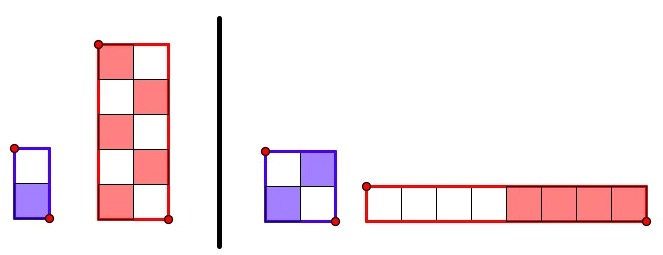# An Equivalent Fractions Game

In my recent posts, I’ve introduced interactive models for comparing fractions and multiplying fractions. To continue the fraction theme, below (and here) is a Web Sketchpad model in which the need for equivalent fractions arises naturally through the rules of a game.

The model displays two arrays. Dragging the four points changes the arrays’ dimensions. The goal is to drag blue squares into the blue array and red squares into the red array so that a specified fraction of each array is shaded.

Below are two solutions to shading half of each array using a total of 6 squares. Notice how students not only reason about fractions to solve the problem but also the dimensions of the arrays. If 5 red squares are to shade half the red array, for example, what dimensions of the array will yield a total of 10 squares?Pressing New Problem changes the total number of required squares. Navigating from page to page with the arrows in the lower-right corner presents problems with different fractions.

As students solve the various challenges, they encounter some interesting complexities. Consider, for example, the goal of shading 2/5 of the blue array and 1/2 of the red array with 13 squares. Students may be tempted to take the simple approach and use 2 blue squares in a 1 x 5 blue array. However, this leaves 11 red squares for the red array, and since each array extends to just 10 x 10, it is not possible to create a red array with 22 squares. Instead, students might place 6 blue squares in a 3 x5 blue array and 7 red squares in a 2 x 7 red array.

An annotated list of all our elementary-themed blog posts is here.